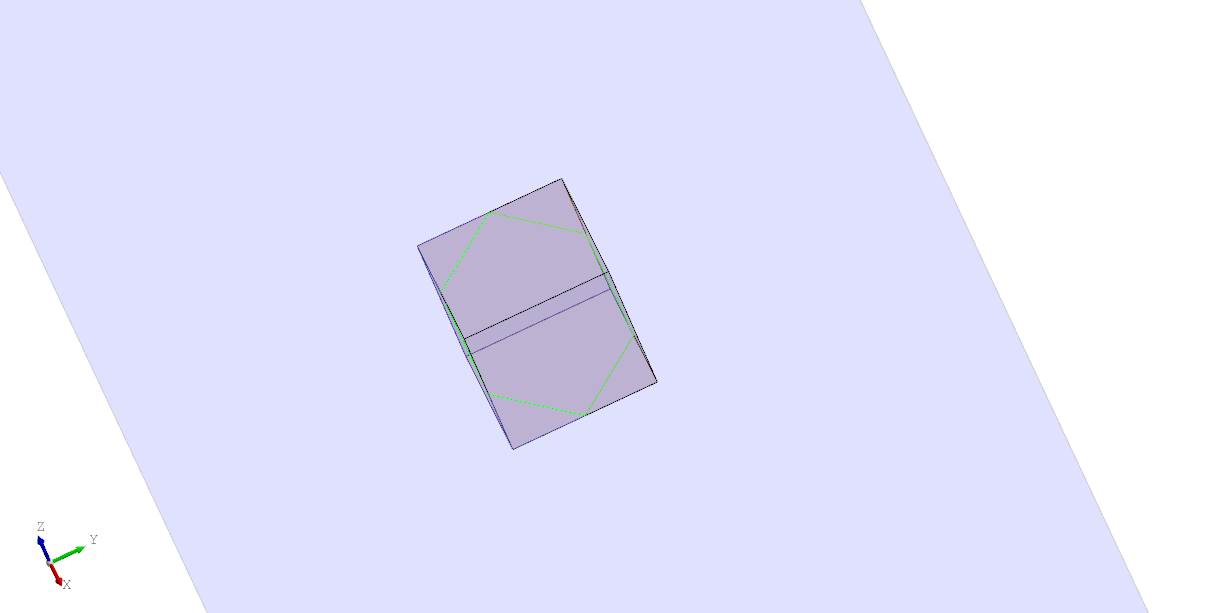The `Intersection` node performs an intersection of two shapes. The shapes can be provided as either `shape1` and `shape2` or as child nodes. If more than two child nodes are used the result will be the intersection of the first with all others. The resulting type depends on the shapes and may be a compound if there are multiple intersections.

For example, the following shows the intersection of an angled plane and a cube. The result is a wire polygon (shown in lime).

``````# Created in DeclaraCAD

enamldef Assembly(Part):
Box: box:
transparency = 0.7
position = (-dx/2, -dy/2, -dz/2)
dx = 3
dy = 3
dz = 3
Plane: plane:
transparency = 0.7
color = 'blue'
direction = (1, 1, 1)
bounds = [(-10, -10), (10, 10)]
Intersection:
color = 'lime'
shape1 = box
shape2 = plane
``````Note: If there is no intersection it will raise an error as the resulting shape is void.

#### Topology intersection

The `Topology` API also provides a shortcut for computing intersections at a lower level. Use `shape1.topology.intersection(shape2)` or `Topology(shape=shape1).intersection(shape2)`. The result is an optional list of TopoDS_Shapes. If there is an error or no intersections it returns an empty list. The intersection node above could be replaced with:

``````        Looper:
iterable = box.topology.intersection(plane) or []
TopoShape:
shape = loop.item
``````